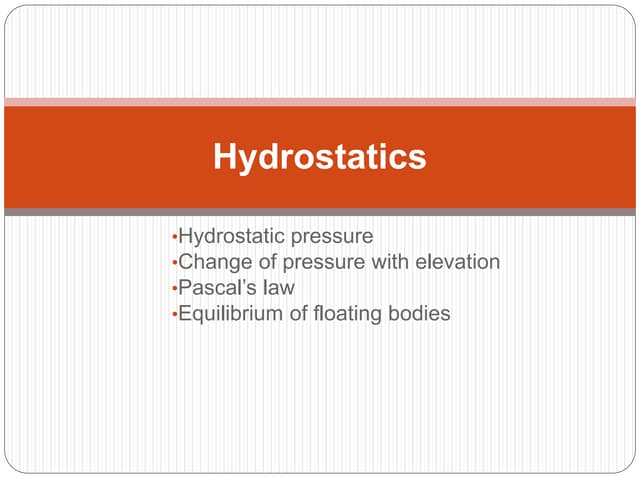Nächste SlideShareHydrostatics Math Problems
1 von 2Hydrostatics Math ProblemsRaha KhanFluidsArjuna Senanayake2018 ii-ing industrial-differential_equation( list-03)-lunesebkcenterFluid Mechanics.pptxJhunLerryTayan3Buoyancy and Archimedes’ PrincpleasifnazarindiaChapter15 ajohn ryan rizal

### An object floats first in fluid of density p(1)-900 kg-m^3- then in a.docx

1. An object floats first in fluid of density p(1)=900 kg/m^3, then in a fluid of density p(2)=1000 kg/m^3, and finally in a fluid of density p(3)=1100 kg/m^3. a) Rank the densities according to the magnitude of the buoyant force on the object, greatest first. b) Rank the densities according to the amount of fluid displaced by the object, greatest first. An object floats first in fluid of density p(1)=900 kg/m^3, then in a fluid of density p(2)=1000 kg/m^3, and finally in a fluid of density p(3)=1100 kg/m^3. a) Rank the densities according to the magnitude of the buoyant force on the object, greatest first. b) Rank the densities according to the amount of fluid displaced by the object, greatest first. a) Rank the densities according to the magnitude of the buoyant force on the object, greatest first. b) Rank the densities according to the amount of fluid displaced by the object, greatest first. Solution a) since the object floats on each liquid hence the amount on buoyant force on object is equal to the wieght of the object in each case which is constant hence buoyant force in each case would be the same, p(1) = p(2) = p(3) but if the same volume of object is dipped inside the liquid in each case then buoyant force would be directly proportional to the densities , p(3)>p(2)>p(1) b) for equal buoyant force = the wieght of the floating object, the volume displaced would be inversely proportional to the density, hence, p(1)>p(2)>p(3).